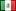Conversions## Metric Conversion Basics

• 1 kilometer (km) = 0.62 mile (mi)
• 1 kilometer (km) = 3280.8 feet (ft)
• 1 meter (m) = 3.28 feet (ft)
• 1 centimeter (cm) = 0.39 inch (in)
• 1 millimeter (mm) = 0.039 inch (in)
• 1 inch (in) = 2.54 centimeters (cm)
• 1 inch (in) = 25.4 millimeters (mm)
• 1 foot (ft) = 0.30 meter (m)
• 1 yard (yd) = 0.91 meter (m)
• 1 yard (yd) = 0.00091 kilometer (km)
• 1 mile (mi) = 1.61 kilometers (km)

The conversion is:

To convert mm to inches, divide by 25.4

To convert inches to mm, multiple by 25.4

The fraction is:

1 kilometer (km) / 0.62 miles (mi)

## Metric Conversion Calculator

Length / Distance Converter
Unit converter for length
 Value From MillimetersCentimetersMetersKilometersFeetInchesYardsMilesDecametersHectometersDecimeters To MillimetersCentimetersMetersKilometersFeetInchesYardsMilesDecametersHectometersDecimeters

## Currency CalculatorMexican Peso Converter